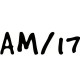Class 10 Maths Notes

# CBSE Class 10 Maths Chapter 6 TrianglesWritten by
TrianglesTriangle

### Triangles

Triangle is derived from word Tri meaning three & Angle, implies 3 angles. It is a closed figure formed by 3 intersecting lines. It has 3 sides, 3 angles, 3 vertices.Triangle is used in various fields
1. Surveyor use it to find height of building
2. It is used in construction of Bridge
3. It is also used to build roof of the house
4. Some Street sign broads are triangular
5. It is used in Astronomy
6. Also used in Carpentry & many other fields.

### Congruent Figures

Congruent means equal in all respect. These figures have same shape & size.
Similar figures have same shape, but size may differ. All congruent figures are similar but vice versa is not true.
Two polygons of the same number of sides are similar, if
• their corresponding angles are equal and
• Their corresponding sides are in the same ratio (or proportion).
The same ratio of the corresponding sides is referred to as the scale factor for the polygons. World maps and blue prints for the construction of a building are prepared using a suitable scale factor.

### Similarity of Triangles

Two triangles are similar, if
• their corresponding angles are equal Or
• Their corresponding sides are in the same ratio (or proportion).
Both mean same things. If one is true, other has to be true.If corresponding angles of two triangles are equal, then they are known as equiangular triangles.
Thales theorem: The ratio of any two corresponding sides in two equiangular triangles is always the same.
Theorem 1 : If a line is drawn parallel to one side of a triangle to intersect the other two sides in distinct points, the other two sides are divided in the same ratio.Converse Theorem 2: If a line divides any two sides of a triangle in the same ratio, then the line is parallel to the third side.### Criteria for Similarity of Triangles

The symbol ~ stands for ‘ is similar to’. As done in the case of congruency of two triangles, the similarity of two triangles should also be expressed symbolically, using correct correspondence of their vertices. For example, for the triangles ABC and DEF, we cannot write Δ ABC ~ Δ EDF or Δ ABC ~ Δ FED. However, we can write Δ BAC ~ Δ EDF.Two triangles are similar, if
• their corresponding angles are equal or
• their corresponding sides are in the same ratio (or proportion).
That is, Δ ABC and Δ DEF are similar , if
(i) ∠ A = ∠ D, ∠ B = ∠ E, ∠ C = ∠ F or
(ii) AB/ DE =  CA/FD = BC/EF

Theorem 3: If in two triangles, corresponding angles are equal, then their corresponding sides are in the same ratio (or proportion) and hence the two triangles are similar.
This criterion is referred to as the AAA (Angle–Angle–Angle) criterion of similarity of two triangles.If two angles of one triangle are respectively equal to two angles of another triangle, then the two triangles are similar. This may be referred to as the AA similarity criterion for two triangles.

Theorem 4 : If in two triangles, sides of one triangle are proportional to (i.e., in the same ratio of ) the sides of the other triangle, then their corresponding angles are equal and hence the two triangles are  similiar.This criterion is referred to as the SSS (Side–Side–Side) similarity criterion for two triangles.
Theorem 5 : If one angle of a triangle is equal to one angle of the other triangle and the sides including these angles are proportional, then the two triangles are similar.
This criterion is referred to as the SAS (Side–Angle–Side) similarity criterion for two triangles.

### Areas of Similar Triangles

Theorem 6 : The ratio of the areas of two similar triangles is equal to the square of the ratio of their corresponding sides.

### Pythagoras Theorem

Theorem 7 : If a perpendicular is drawn from the vertex of the right angle of a right triangle to the hypotenuse then triangles on both sides of the perpendicular are similar to the whole triangle and to each other.Pythagoras Theorem 8 : In a right triangle, the square of the hypotenuse is equal to the sum of the squares of the other two sides.Converse Pythagoras Theorem 9 : In a triangle, if square of one side is equal to the sum of the squares of the other two sides, then the angle opposite the first side is a right angle.

### MATHS Revision Notes

Chapter:01  Real Numbers System
Chapter:02  Polynomials
Chapter:03  Pair of Linear Equations in Two Variables
Chapter:05  Arithemetic Progressions
Chapter:06  Triangles
Chapter:07  Coordinate Geometry
Chapter:08  Introduction to Trignometry
Chapter:09  Some Application Of Trignometry
Chapter:10  Circles
Chapter:11  Constructions
Chapter:12  Area Related to Cirles
Chapter:13  Surface Area Volume
Chapter:14  Stastistics
Chapter:15  Probability

Science Revision Notes

English Revision Notes

Economics Revision Notes